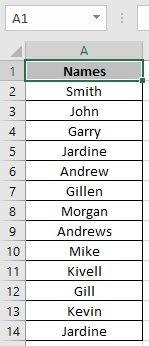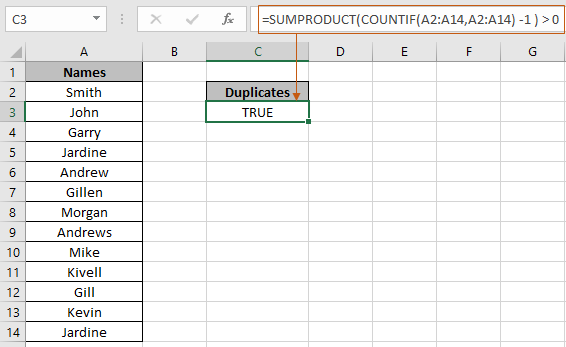# How to Know If Any Duplicates in a Range in Excel

In this article, we will learn about how to know if the list has duplicate values in excel.

When a list contains a lot of values and we need to confirm if the list contains duplicate values or not. For this we will make a combination of excel functions and use the formula to get the desired result. Use the SUMPRODUCT function & COUNTIF function.

SUMPRODUCT function is a mathematical function in Excel. It operates on multiple ranges. It multiplies the corresponding arrays and then adds them.

Syntax:

= SUMPRODUCT ( list1, [list2], … )

COUNTIF function of excel just counts the number of cells with a specific condition in a given range.

Syntax:

= COUNTIF ( list, condition )

Formation of the formula:
First we need to check every element of the list with every other element of the same list. Then we will set a limit on the count by subtracting 1 from each occurrence and returns the required result.

= SUMPRODUCT ( COUNTIF ( list, list ) -1 ) > 0

Let’s understand this formula using in the example shown below.
For this example, we took some names as a list in excel as shown below.We need to find out if there's a duplicate value or not.
Use the Formula

= SUMPRODUCT ( COUNTIF( A2:A14 , A2:A14 ) -1 ) > 0

Explanation :
COUNTIF function counts the occurrence of each value in the list and returns an array of numbers, where number represents their counts

{ 1 ; 1 ; 1 ; 2 ; 1 ; 1 ; 1 ; 1 ; 1 ; 1 ; 1 ; 1 ; 2 }
Subtract 1 from each value in the list.

{ 0 ; 0 ; 0 ; 1 ; 0 ; 0 ; 0 ; 0 ; 0 ; 0 ; 0 ; 0 ; 1 }

Now the SUMPRODUCT function adds up all the value and returns TRUE if the result is greater than 0 or else it returns FALSE.In the above snapshot the formula is used to see if there is any duplicate value in the list.
Blank cells in the list may cause the formula to generate incorrect results. To ignore the blank cells in the list, use the formula below.

Use this formula if the list contains blank cells

= SUMPRODUCT ( ( COUNTIF ( list , list ) - 1 ) * ( list < > " " ) ) > 0

Hope you understand  how to see if the list has duplicate values in Excel. Explore more articles on Mathematical formulation like Increase by percentage and Profit margin percentage in Excel here. Mention your queries in the comment box below. We will help you with it.

Related Articles

Highlight Duplicates Values Among Two or More Columns

Count Unique Values In Excel

How to use the SUMPRODUCT function

How to use the COUNTIF function

Popular Articles

Edit a dropdown list

If with conditional formatting

If with wildcards

Vlookup by date

Terms and Conditions of use

The applications/code on this site are distributed as is and without warranties or liability. In no event shall the owner of the copyrights, or the authors of the applications/code be liable for any loss of profit, any problems or any damage resulting from the use or evaluation of the applications/code.